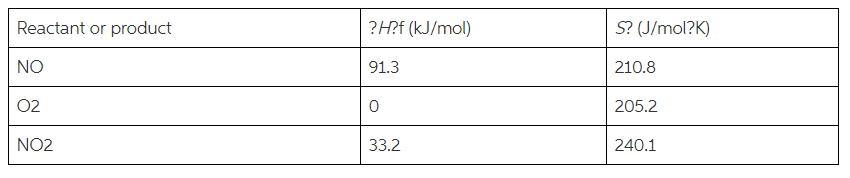Problem: Consider the oxidation of NO to NO 2 :NO(g) + 1/2O2(g)→NO2(g).Calculate ΔG°rxn at 25°C.Express your answer with the appropriate units. Determine whether the reaction is spontaneous at standard conditions.

FREE Expert Solution
88% (413 ratings)
Problem Details

Consider the oxidation of NO to NO 2 :
NO(g) + 1/2O2(g)→NO2(g).

Calculate ΔG°rxn at 25°C.Express your answer with the appropriate units. Determine whether the reaction is spontaneous at standard conditions.What scientific concept do you need to know in order to solve this problem?

Our tutors have indicated that to solve this problem you will need to apply the Gibbs Free Energy concept. You can view video lessons to learn Gibbs Free Energy Or if you need more Gibbs Free Energy practice, you can also practice Gibbs Free Energy practice problems .

What is the difficulty of this problem?

Our tutors rated the difficulty of Consider the oxidation of NO to NO 2 :NO(g) + 1/2O2(g)→NO2(g... as medium difficulty.

How long does this problem take to solve?

Our expert Chemistry tutor, Sabrina took 10 minutes to solve this problem. You can follow their steps in the video explanation above.

What professor is this problem relevant for?

Based on our data, we think this problem is relevant for Professor Goodman's class at MONTANA.